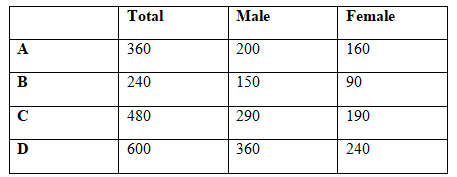# SBI Clerk Prelims 2021 Quantitative Aptitude Questions (Day-18)

Dear Aspirants, Our IBPS Guide team is providing new series of Quantitative Aptitude Questions for SBI Clerk Prelims 2020 so the aspirants can practice it on a daily basis. These questions are framed by our skilled experts after understanding your needs thoroughly. Aspirants can practice these new series questions daily to familiarize with the exact exam pattern and make your preparation effective.

Start Quiz

Missing number series

Directions (01-05): What value should come in the place of (?) in the following number series?

1) 2190, 2736, 3366, 4086,?, 5820

A.4109

B.4516

C.4782

D.4771

E.4902

2) 3, 28, 197, 986, 2959,?

A.2960

B.2970

C.3190

D.2280

E.3280

3) 17, 25, 150, 662, 1993, 4737,?

A.8990

B.9170

C.7370

D.8620

E.9650

4) 24, 24, 36, 72, 180,?

A.280

B.480

C.360

D.540

E.320

5) 1260, 210, 42, 10.5, 3.5,?

A.1.75

B.1.25

C.2.25

D.1.60

E.2.75

Caselet

Directions (06-10): Study the following information carefully and answer the questions given below.

The number of employees from A is 50% more than the number of employees in B. The number of employees from B is half of the number of employees from C and the number of employees from D is 25% more than the number of employees from C. The number of male to female employees to from D in the ratio of 3: 2 and the ratio of the number of female employees from B is 40% less than the number of male employees from B. The number of male employees from A is 50 more than the number of male employees from B. The number of female employees from C is 100 more than the number of female employees from B. Total number of employees from A, C and D is 1440.

6) What is the average number of female employees in all the companies together?

A.120

B.140

C.160

D.170

E.180

7) Quantity I:
Sum of the male employees in A and B.

Quantity II: Number of male employees in D.

A.Quantity I > Quantity II

B.Quantity I ≥ Quantity II

C.Quantity I = Quantity II

D.Quantity I < Quantity II

E.Quantity I ≤ Quantity II

8) What is the difference between the number of male employees in C and the number of female employees in A?

A.110

B.120

C.130

D.140

E.None of these

9) Total number of male employee in A and D together is approximately what percent more than the total number of employees in C?

A.14.48%

B.16.67%

C.18.89%

D.33.33%

E.None of these

10) What is the ratio of the total number of male to female employees from all the companies together?

A.25: 17

B.24: 19

C.23: 16

D.22: 15

E.None of these

133 – 7 = 2190

143 – 8 = 2736

153 – 9 = 3366

163 – 10 = 4086

173 – 11 = 4902

183 – 12 = 5820

3 * 9 + 1 = 28

28 * 7 + 1 = 197

197 * 5 + 1 = 986

986 * 3 + 1 = 2959

2959 * 1 + 1 = 2960

17 + 23 = 25

25 + 53 = 150

150 + 83 = 662

662 + 113 = 1993

1993 + 143 = 4737

4737 + 173 = 9650

24 * 1 = 24

24 * 1.5 = 36

36 * 2 = 72

72 * 2.5 = 180

180 * 3 = 540

1260 ÷ 6 = 210

210 ÷ 5 = 42

42 ÷ 4 = 10.5

10.5 ÷ 3 = 3.5

3.5 ÷ 2 = 1.75

Directions (6-10) :

Number of employees from B = x

Number of employees from A = x * 150/100 = 3x/2

Number of employees from C = 2x

Number of employees from D = 2x * 125/100 = 5x/2

(3x/2 + 2x + 5x/2) = 1440

12x = 1440 * 2

X = 240

Total number of employees in A = 3/2 * 240 = 360

Total number of employees in D = 5/2 * 240 = 600

Total number of employee in C = 2 * 240 = 480

Total number of employee in B = 240

Number of female employees in D = 2y

Number of male employees in D = 3y

2y + 3y = 600

5y = 600

Y = 120

Number of male employees in D = 3 * 120 = 360

Number of female employees in D = 120 * 2 = 240

Number of male employees in B = z

Number of female employees in B = z * 60/100

z + 3z/5 = 240

8z = 240 * 5

Z= 150

Number of female employees in B = 150 * 60/100 = 90

Number of male employees in A = 150 + 50 = 200

Number of female employees in A = 360 – 200 = 160

Number of female employees in C = 100 + 90 =190

Number of male employees in C = 480 – 190 = 290Average number of female employees = (160 + 90 + 190 + 240)/4 = 170

From Quantity I,

Required Sum = 200 + 150 = 350

From Quantity II,

Number of male employees in D = 360

Quantity I < Quantity II

Difference = 290 – 160 = 130

Required percentage = ((200 + 360) – 480)/480 * 100 = 16.67%

Required ratio = (200 + 360 + 150 + 290): (160 + 90 + 190 + 240)

= 1000: 680

= 25: 17

 Check Here to View SBI Clerk Prelims 2021 Quantitative Aptitude Questions Day – 17 Day – 16 Day – 15
3 1 vote
Rating